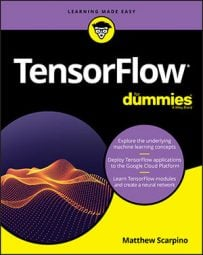##### TensorFlow For DummiesMost of the mathematical routines accept floating-point values as input and return floating-point values as output. But many applications need to convert floating-point values into integer values. For this reason, TensorFlow provides the rounding operations listed in the following table.

Rounding and Comparison Operations

 Function Description `round(x, name=None)` Rounds to the nearest integer, rounding up if there are two nearest integers `rint(x, name=None)` Rounds to the nearest integer, rounding to the nearest even integer if there are two nearest integers `ceil(x, name=None)` Returns the smallest integer greater than the value `floor(x, name=None)` Returns the greatest integer less than the value `maximum(x, y, name=None)` Returns a tensor containing the larger element of each input tensor `minimum(x, y, name=None)` Returns a tensor containing the smaller element of each input tensor `argmax(x, axis=None, name=None, dimension=None)` Returns the index of the greatest element in the tensor `argmin(x, axis=None, name=None, dimension=None)` Returns the index of the smallest element in the tensor
The table also lists functions that perform comparisons. These functions return maximum and minimum values, both within a tensor and across two tensors.

The `round `function examines each element of a tensor and returns the closest integer. If two closest integers are equally close, it returns the one further from zero. `rint `is similar, but rounds to the nearest even value. The following code demonstrates how you can use `round`, `rint`, `ceil`, and `floor`:

`t = tf.constant([-6.5, -3.5, 3.5, 6.5])r1 = tf.round(t)                 # [-6. -4. 4. 6.]r2 = tf.rint(t)                  # [-6. -4. 4. 6.]r3 = tf.ceil(t)                  # [-6. -3. 4. 7.]r4 = tf.floor(t)                 # [-7. -4. 3. 6.]`
The next two functions in the table, `maximum `and `minimum`, are easy to understand. `maximum `returns a tensor containing the larger element of each input tensor, and `minimum `returns a tensor containing the smaller element of each input tensor.

`argmax `and `argmin `return the index values of the largest and smallest elements of a tensor. The following code shows how you can use these functions:

`t1 = tf.constant([0, -2, 4, 6])t2 = tf.constant([[1, 3], [7, 2]])r1 = tf.argmin(t1)                      # 1r2 = tf.argmax(t2)                      # [ 1 0 ]`
If a tensor has multiple maximum/minimum values, `argmax `and `argmin `will return the index values of the first occurring element.